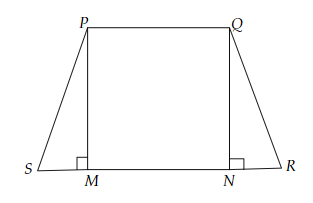# If the non-parallel sides of a trapezium are equal, prove that it is cyclic.

Given:

The non-parallel sides of a trapezium are equal.

To do:

We have to prove that it is cyclic.

Solution:Let $PQRS$ be a trapezium in which $PQ \| RS$ and $PS=QR$

Draw $PM \perp RS$ and $QN \perp RS$

In $\triangle PSM$ and $\triangle QRN$,

$PS=QR$

$\angle PMS=\angle QNR=90^o$

$PM=QN$  (Perpendicular distance between two parallel lines is equal)

Therefore, by RHS congruency,

$\triangle PMS \cong QNR$

This implies,

$\angle PSR=\angle QRS$.........(i)    (CPCT)

$\angle QPS$ and $\angle PSR$ are on the same side of the transversal $PS$

$\angle QPS+\angle PSR=180^o$

$\angle QPS+\angle QRS=180^o$        [From (i)]

This implies,

Opposite angles are supplementary.

Hence, the given trapezium is cyclic.

Updated on: 10-Oct-2022

46 Views